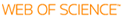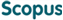### BROWSE

#### Related ResearcherLee, Chang Hyeong
Stochastic Analysis & Simulation(SAS) Lab
Research Interests
• Stochastic analysis/computation, epidemic modeling, biological system simulation

#### ITEM VIEW & DOWNLOAD

Solution Interpolation Method for Highly Oscillating Hyperbolic Equations

Cited 0 times inCited 0 times inTitle
Solution Interpolation Method for Highly Oscillating Hyperbolic Equations
Author
Issue Date
2013-11
Publisher
HINDAWI PUBLISHING CORPORATION
Citation
JOURNAL OF APPLIED MATHEMATICS, v.2013, pp.546031
Abstract
This paper deals with a novel numerical scheme for hyperbolic equations with rapidly changing terms. We are especially interested in the quasilinear equation u(t) + au(x). = f(x)u + g(x)u(n) and the wave equation u(tt) = f(x)u(xx) that have a highly oscillating term like f(x) = sin(x/epsilon), epsilon << 1. It also applies to the equations involving rapidly changing or even discontinuous coefficients. The method is based on the solution interpolation and the underlying idea is to establish a numerical scheme by interpolating numerical data with a parameterized solution of the equation. While the constructed numerical schemes retain the same stability condition, they carry both quantitatively and qualitatively better performances than the standard method.
URI
https://scholarworks.unist.ac.kr/handle/201301/2850
URL
https://www.hindawi.com/journals/jam/2013/546031/
DOI
10.1155/2013/546031
ISSN
1110-757X
Appears in Collections:
MTH_Journal Papers
Files in This Item:
546031.pdf Download

can give you direct access to the published full text of this article. (UNISTARs only)Items in DSpace are protected by copyright, with all rights reserved, unless otherwise indicated.